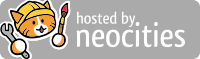### Simple power calcualtor!

Simply input Watts of the appliance. How many hours every day you use it. For how many days do you use the appliance [For example 24 days in a month]. Press calculate to show the Units it will cosume.

You can change the cost of unit and press Calculate Cost to get whats its going to cost you approximately. Its easy to get cost per unit. Take your electricity bill. It will have units you have consumed and the total bill amount for them. Just device total Amount of bill by Units consumed and you will get cost per unit.

For example if I have a wifi modem and router of 15 Watt running 24 hours every day for whole monnth that is 30 days then my Unit consumption per 30 days will be 10.8. And if my cost per Unit is 9 cents then it will cost me 97.2 cents every month.

These are very rough and simple calculation just to give you an idea of the cost involved and not at all accurate !

 Watts Hours Days Units [Kwh] Cost per Unit Cost